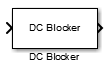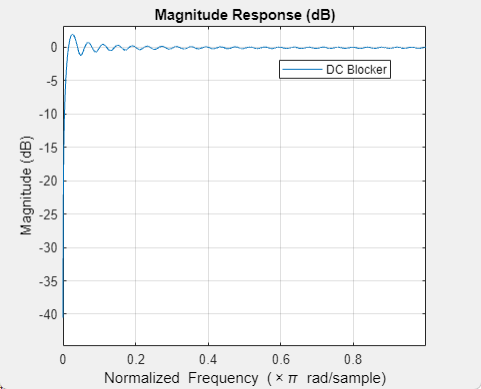# DC Blocker

Block DC component

•Libraries:
DSP System Toolbox / Signal Operations
Communications Toolbox / Comm Filters
Communications Toolbox / RF Impairments Correction
DSP System Toolbox HDL Support / Signal Operations
Communications Toolbox HDL Support / Comm Filters

## Description

The DC Blocker block removes the DC component of the input signal.

This block supports C/C++ code generation and SIMD code generation. For details, see Code Generation.

## Ports

### Input

expand all

Specify the input signal that contains the DC component as a scalar, vector, or a matrix. When the input is fixed-point, it is signed only.

Data Types: `single` | `double` | `int8` | `int16` | `int32` | `fixed point`
Complex Number Support: Yes

### Output

expand all

Output signal with the DC component removed. When the output is fixed-point, it is signed only.

The size, data type, and complexity of the output signal are the same as that of the input signal.

Data Types: `single` | `double` | `int8` | `int16` | `int32` | `fixed point`
Complex Number Support: Yes

## Parameters

expand all

Specify the algorithm used for estimating the DC offset. Select from the following:

• `IIR` uses a recursive estimate based on a narrow, lowpass elliptic filter. This algorithm typically uses less memory than FIR and is more efficient.

• `FIR` uses a nonrecursive, moving-average estimate. This algorithm typically uses more memory than IIR and is less efficient.

• `CIC` uses a lowpass filter that does not employ any multipliers. If the algorithm is `CIC`, then fixed-point data must be input to the DC Blocker.

• `Subtract mean` computes the means of the columns of the input matrix and subtracts the means from the input. This method does not retain state between inputs. For example, if the input is `[1 2 3 4; 3 4 5 6]`, then the DC Blocker block in ```Subtract mean``` mode outputs ```[-1 -1 -1 -1; 1 1 1 1]```.

Specify the normalized filter bandwidth as a real scalar greater than 0 and less than 1.

#### Dependencies

The DC Blocker uses this parameter only when the estimation algorithm is set to `IIR` or `CIC`.

Data Types: `single` | `double` | `int8` | `int16` | `int32` | `int64` | `uint8` | `uint16` | `uint32` | `uint64`

Specify the filter order of the lowpass IIR elliptic filter as an integer greater than 3.

#### Dependencies

The DC Blocker uses this parameter only when the estimation algorithm is set to `IIR`.

Data Types: `single` | `double` | `int8` | `int16` | `int32` | `int64` | `uint8` | `uint16` | `uint32` | `uint64`

Specify, as a positive integer, the number of samples to use when the estimation algorithm is set to `FIR`.

#### Dependencies

The DC Blocker uses this parameter only when the estimation algorithm is set to `FIR`.

Data Types: `single` | `double`

Opens the `fvtool` and displays the magnitude response of the DC Blocker. The response is based on the block parameters. Changes made to these parameters update `fvtool`.To update the magnitude response while `fvtool` is running, modify the block parameters and click .

Specify the type of simulation to run as one of the following:

• `Code generation` –– Simulate model using generated C code. The first time you run a simulation, Simulink® generates C code for the block. Simulink reuses the C code in subsequent simulations as long as the model does not change. This option requires additional startup time but subsequent simulations are faster compared to ```Interpreted execution```.

• `Interpreted execution` –– Simulate model using the MATLAB®  interpreter. This option shortens startup time but subsequent simulations are slower compared to ```Code generation```.

## Block Characteristics

 Data Types `double` | `fixed point` | `integer` | `single` Direct Feedthrough `no` Multidimensional Signals `no` Variable-Size Signals `yesa` Zero-Crossing Detection `no`

## Algorithms

expand all

The DC blocker subtracts the DC component from the input signal. You can estimate the DC component by using the IIR, FIR, CIC, or subtract mean algorithm.

 Nezami, M.K. “Performance Assessment of Baseband Algorithms for Direct Conversion Tactical Software Defined Receivers: I/Q Imbalance Correction, Image Rejection, DC Removal, and Channelization.” In MILCOM 2002. Proceedings, 369–76. Anaheim, CA, USA: IEEE, 2002.